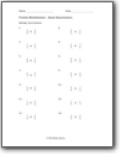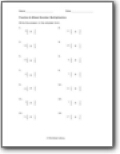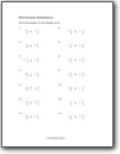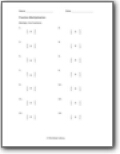# Fraction Multiplication Worksheets

#### How to Multiply Fractions

The numbers starting from zero are whole numbers. Then there are fractions. These represent the portion of a whole number. There are two types of fractions; proper fractions and improper fractions. Proper fractions are the ones where the denominator is greater than the numerator. The value of these fractions lies between 0 and 1. The improper fractions are those where the denominator is less than the numerator. The value of these fractions is greater than 1. Multiplying fractions is pretty simple. All you have to do is multiply the numerator with the numerator and multiply the denominators and achieve a single fraction. For example, you are asked to multiply these two fractions; a/b and c/d. You have to multiply the numerators of both the fractions together and multiply the denominators of both fractions together. The final fraction becomes; ac/bd.###### Same Denominators

We start at the beginning by finding the product of like fractions.###### Fraction & Mixed Number Multiplication

You will multiply a mixed number with a fraction. The denominators are all over the place.###### Mixed Number Multiplication

You will process a product operation between a mixed number by another mixed number. A go way to approach these problems is to start by converting everything to a simple fraction.###### Fraction Multiplication

The denominators vary across these products. They can be like or unlike.